# Understanding Optical Lens Geometries

Optical lenses are the most important tools in optical design for controlling light. When optical designers talk about optical lenses, they are either referring to a single lens element or an assembly of lens elements (Figure 1). Examples of single elements are plano-convex (PCX) lenses, double-convex (DCX) lenses, aspheric lenses, etc; examples of assemblies of elements are telecentric imaging lenses, infinity-corrected objectives, beam expanders, etc. Each combination is comprised of a series of lens elements and each element has a specific lens geometry which controls light in its own way.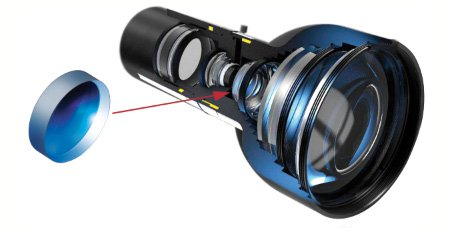## SNELL'S LAW OF REFRACTION

Before delving into each type of lens geometry, consider how optical lenses bend light using the property of refraction. Refraction is the means by which light is deviated by a certain amount when it enters or leaves a medium. This deviation is a function of the index of refraction of the medium and the angle the light makes with respect to the surface normal. This property is governed by Snell's Law of Refraction (Equation 1) where $\small{n_1}$ is the index of the incident medium, $\small{\theta_1}$ is the angle of the incident ray, $\small{n_2}$ is the index of the refracted medium, and $\small{\theta_2}$ is the angle of the refracted ray. Snell's Law describes the relationship between the angles of incidence and transmission when a ray travels between multiple media (Figure 2).

(1)$$n_1 \, \sin{\left( \theta_1 \right)} = n_2 \, \sin{\left( \theta_2 \right)}$$## OPTICAL LENS TERMINOLOGY

All optical lenses obey Snell's Law of Refraction. Consequently, it is the optical lens geometry (i.e. the surface profile) that determines how light behaves as it propagates through the optical element. To understand the terminology used in optical lens specifications, consider 10 common terms (Table 1). For more detailed definitions and a list of additional terms, please view our Glossary.

Abbreviation Terminology – Definition Common Optical Lens Terminology $\small{D}$, Dia. Diameter – The physical size of a lens. $\small{R, R_1, R_2}$ , etc. Radius of Curvature – The directed distance from the vertex of a surface to the center of curvature. $\small{\text{EFL}}$ Effective Focal Length – An optical measurement given as the distance from a principal plane of an optical lens to its imaging plane. $\small{\text{BFL}}$ Back Focal Length – A mechanical measurement given as the distance between the last surface of an optical lens to its image plane. P, P" Principle Plane – A hypothetical plane where incident light rays can be considered to bend due to refraction. EFL is specified from a principal plane location. $\small{\text{CT, CT}_1, \text{CT}_2}$, etc. Center Thickness – The distance from a primary principal plane location to the end of an element. $\small{\text{ET}}$ Edge Thickness – A calculated value that depends on radii, diameter, and center thickness of a lens. $\small{d_b}$ Entrance Beam Diameter – Diameter of collimated light entering an axicon. $\small{d_r}$ Exit Beam Diameter – Diameter of ring of light exiting an axicon. $\small{L}$ Length – The physical distance from end to end of a cylindrical element (e.g. cylinder lens) or the distance from apex to workpiece of an axicon.

## OPTICAL LENS GEOMETRIES

Using the common terminologies from Table 1, it is easy to understand technical figures for each type of single lens element. Table 2 shows 10 of the most commonly used optical lenses and their typical applications. As optical technology advances, additional single-lens geometries such as focus-tunable lenses and assemblies such as telecentric lenses are becoming valuable tools for optical design. To learn more about telecentric lenses, view The Advantages of Telecentricity.

 Common Optical Lens Geometries Plano-Convex (PCX) Lens | View ProductIdeal for collimation and focusing applications utilizing monochromatic illumination. Note: Orient the curved surface of a PCX lens towards the source for optimal performance.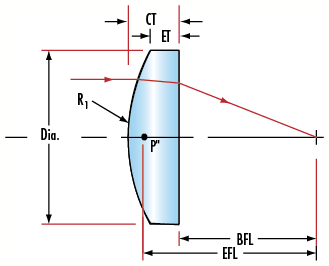Double-Convex (DCX) | View ProductIdeal for image relay, and for imaging of objects at close conjugates. Note: Aberrations will increase as the conjugate ratios increase. Also known as biconvex lenses.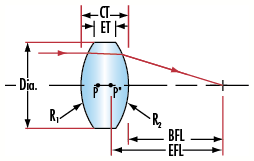Plano-Concave (PCV) Lens | View Product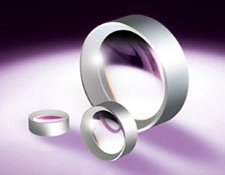Comprised of one flat and one inward curved surface. Ideal for beam expansion, light projection, and expanding the focal length of an optical system.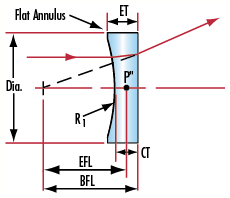Double-Concave (DCV) Lens | View Product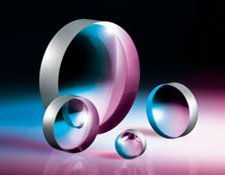Comprised of two inward, equally curved surfaces. Ideal for beam expansion, light projection, and expanding the focal length of an optical system. Also known as biconcave lenses.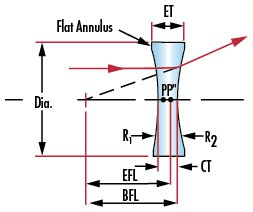Positive Achromatic Lens | View ProductPerforms similar function as a PCX or DCX lens, but can provide smaller spot sizes and superior image quality. Achromatic lenses are useful for reducing spherical and chromatic aberration. Negative version for diverging light as available. For additional information, view Why Use an Achromatic Lens?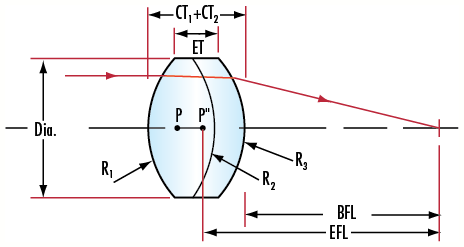Aspheric Lens | View ProductIdeal for laser focusing or for replacing multiple spherical lens elements in a system. Useful for eliminating spherical aberration and greatly reducing other aberrations. For additional information, view All About Aspheric Lenses.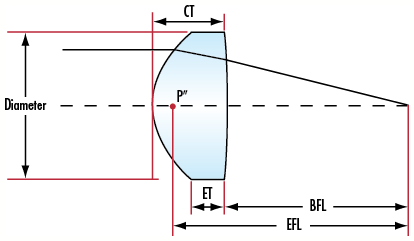Positive Cylinder Lens | View ProductIdeal for focusing incoming light to a line or to change the aspect ratio of an image. Negative version also available.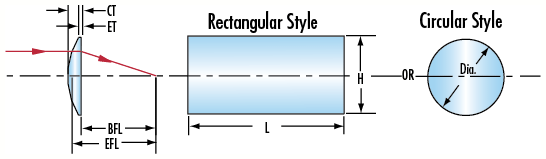Plano-Convex (PCX) Axicons | View Product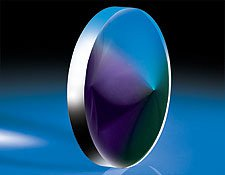Ideal for focusing laser light into a ring with a constant thickness. Note: A smaller apex angle generates a larger ring. For additional information, view An In-Depth Look at Axicons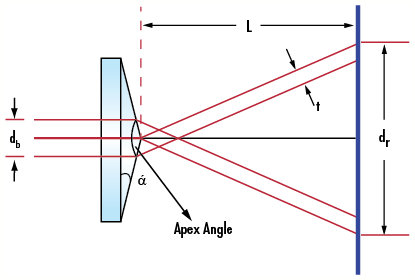(Full) Ball Lens | View ProductIdeal for fiber coupling, endoscopy, and barcode scanning applications. Half ball lens version also available. For additional information, view Understanding Ball Lenses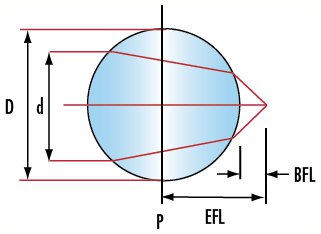Rod Lens | View ProductIdeal for fiber coupling and endoscopy applications. 45° version also available.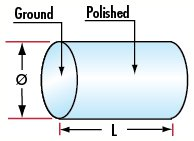Optical lenses come in many shapes and sizes – from plano-convex (PCX) to aspheric. Knowing the advantages and disadvantages of each lens type is crucial when choosing between optics as each has its own purpose. Understanding optical lens geometries helps anyone, from novice to expert, choose the best optical lens in any optical design.